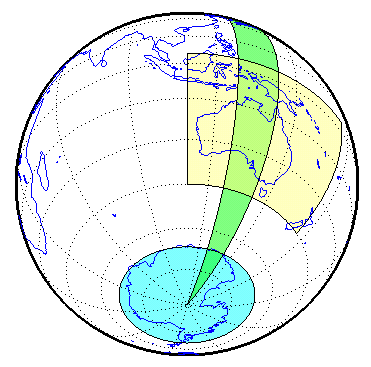Documentation

## Syntax

```[lat,lon] = outlinegeoquad(latlim,lonlim,dlat,dlon) ```

## Description

`[lat,lon] = outlinegeoquad(latlim,lonlim,dlat,dlon)` constructs a polygon that traces the outline of the geographic quadrangle defined by `latlim` and `lonlim`. Such a polygon can be useful for displaying the quadrangle graphically, especially on a projection where the meridians and/or parallels do not project to straight lines. `latlim` is a two-element vector of the form: `[southern-limit northern-limit]` and `lonlim` is a two-element vector of the form: `[western-limit eastern-limit]`. `dlat` is a positive scalar that specifies a minimum vertex spacing in degrees to be applied along the meridians that bound the eastern and western edges of the quadrangle. Likewise, `dlon` is a positive scalar that specifies a minimum vertex spacing in degrees of longitude to be applied along the parallels that bound the northern and southern edges of the quadrangle. The outputs `lat` and `lon` contain the vertices of a simple closed polygon with clockwise vertex ordering.

## Examples

Display the outlines of three geographic quadrangles having very different qualities on top of a simple base map:

```figure('Color','white') axesm('ortho','Origin',[-45 110],'frame','on','grid','on') axis off load coastlines geoshow(coastlat, coastlon) % Quadrangle covering Australia and vicinity [lat, lon] = outlinegeoquad([-45 5],[110 175],5,5); geoshow(lat,lon,'DisplayType','polygon','FaceAlpha',0.5); % Quadrangle covering Antarctic region antarcticCircleLat = dms2degrees([-66 33 39]); [lat, lon] = outlinegeoquad([-90 antarcticCircleLat], ... [-180 180],5,5); geoshow(lat,lon,'DisplayType','polygon', ... 'FaceColor','cyan','FaceAlpha',0.5); % Quadrangle covering nominal time zone 9 hours ahead of UTC [lat, lon] = outlinegeoquad([-90 90], 135 + [-7.5 7.5], 5, 5); geoshow(lat,lon,'DisplayType','polygon', ... 'FaceColor','green','FaceAlpha',0.5);```## Tips

All input and output angles are in units of degrees. Choose a reasonably small value for `dlat` (a few degrees, perhaps) when using a projection with curved meridians or curved parallels.

To avoid interpolating extra vertices along meridians or parallels, set `dlat` or `dlon` to a value of `Inf`.

### Special Cases

The insertion of additional vertices is suppressed at the poles (that is, if `latlim(1) == -90` or ```latlim(2) == 90```. If `lonlim` corresponds to a quadrangle width of exactly 360 degrees (`lonlim == [-180 180]`, for example), then it covers a full latitudinal zone and includes two separate, NaN-separated parts, unless either

• `latlim(1) == -90` or ```latlim(2) == 90```, so that only one part is needed—a polygon that follows a parallel clockwise around one of the poles.

• `latlim(1) == -90` and ```latlim(2) == 90```, so that the quadrangle encompasses the entire planet. In this case, the quadrangle cannot be represented by a latitude-longitude polygon, and an error results.